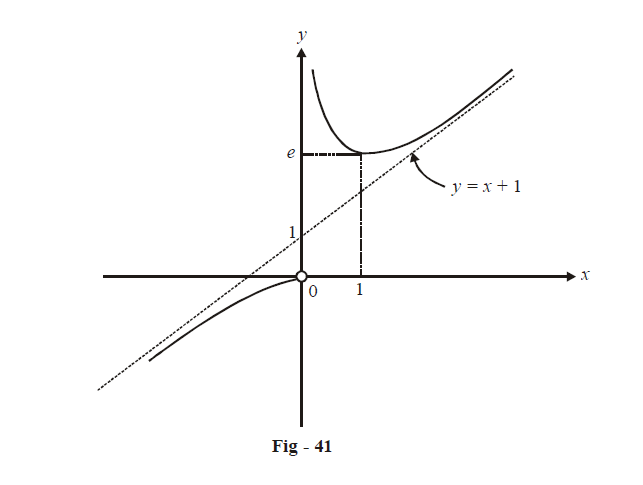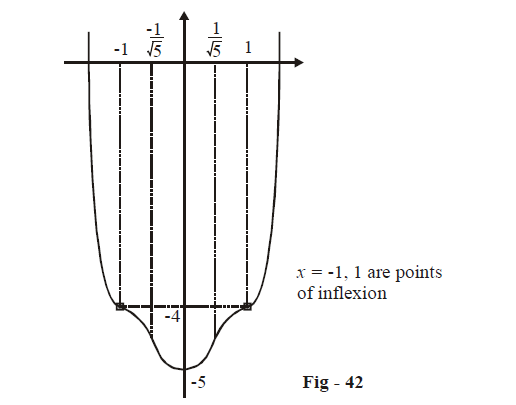# Examples on Graphing Using Derivatives Set 2

Go back to  'Applications of Derivatives'

Example - 37

Plot the graph of $$f\left( x \right) = x{e^{1/x}}$$

Solution: As far as possible, we will try to stick to the general sequence mentioned above for analysing any given function.

* The domain of $$f\left( x \right){\text{is}}\,\mathbb{R}\backslash \left\{ 0 \right\}$$.

* Also, $$f\left( x \right)$$ is continuous and differentiable on $$\mathbb{R}\backslash \{ 0\}$$.

* Now, $$\mathop {\lim }\limits_{x \to {0^ + }} \left( {f\left( x \right)} \right) = \infty .$$ Hence, x = 0 is a vertical asymptote to f (x).

$$\mathop {\lim }\limits_{x \to {0^ - }} \left( {f\left( x \right)} \right) = 0.$$

\begin{align}\mathop {\lim }\limits_{x \to \pm \infty } \left( {\frac{{f\left( x \right)}}{x}} \right) = \mathop {\lim }\limits_{x \to \pm \infty } \left( {{e^{\frac{1}{x}}}} \right) = 1\end{align}

Also,   $$\mathop {\lim }\limits_{x \to \pm \infty } \left( {f\left( x \right) - x} \right) = \mathop {\lim }\limits_{x \to \pm \infty } x\left( {{e^{\frac{1}{x}}} - 1} \right)$$

\begin{align}&= \mathop {\lim }\limits_{y \to 0} \frac{{{e^y} - 1}}{y}\,\,\,\,\,\,\,\left( {{\rm{where}}\,y = \frac{1}{x}} \right)\\ &= 1\end{align}

$$\Rightarrow \quad y = x + {\rm{ }}1$$ is an inclined asymptote to f(x).

* Quoted from I.A. Maron: Problems in Calculus

\begin{align} &* \qquad f'\left( x \right) = x{e^{1/x}} \cdot \left( {\frac{{ - 1}}{{{x^2}}}} \right) + {e^{1/x}}\\&\qquad\quad\qquad = {e^{1/x}}\left( {1 - \frac{1}{x}} \right)\\&\;\;\qquad f'\left( x \right) > 0\,\,\,{\rm{for}}\,\,\,x \in \left( { - \infty ,0} \right) \cup \left( {1,\infty } \right)\\&\;\;\qquad f'\left( x \right) < 0\,\,\,{\rm{for}}\,\,\,x \in \left( {0,1} \right)\\&\;\;\qquad f'\left( x \right) = 0\,\;{\rm{for}}\,\;x = 1;\,\ \text{ a local minimum point;} f(1) = e \end{align}Example - 38

Sketch the graph of $$f\left( x \right) = {x^6} - 3{x^4} + 3{x^2} - 5$$.

Solution:

*          The domain is obviously $$\mathbb{R}$$

*          (x) is an even function

*         Since (x) is a polynomial function, it is continuous and differentiable on $$\mathbb{R}$$.

*         It is obvious that there are no asymptotes to (x)

\begin{align}&*\qquad f'\left( x \right) = 6{x^5} - 12{x^3} + 6x\\ & \qquad\qquad\quad\; = 6x\left( {{x^4} - 2{x^2} + 1} \right)\\ &\qquad\qquad\quad\; = 6x{\left( {{x^2} - 1} \right)^2}\\&\qquad \;\;\;f'\left( x \right) = 0\,\,{\rm{for}}\,\,x = 0, \pm 1\\ & \qquad\qquad\quad\;= \left( {{x^2} - 1} \right)\left\{ {6\left( {{x^2} - 1} \right) + 24{x^2}} \right\}\\& \qquad\qquad\quad\; = \left( {{x^2} - 1} \right)\left( {30{x^2} - 6} \right) \ldots \ldots \ldots \ldots(1)\\ &\qquad\qquad\quad\; = 6\left( {5{x^4} - 6{x^2} + 1} \right) \\&\qquad\;\;\;\ f\left( 0 \right) = 6,\,\,f\left( { \pm 1} \right) = 0 \end{align}

$$\Rightarrow \quad x = {\rm{ }}0$$ is a point of local minimum and $$x = \pm 1$$ are points of inflexion (verify that f''(x) does not change sign as x crosses $$\pm 1$$).

Now, $$f'\left( x \right) > 0\,\,{\rm{if}}\,\,x > 0$$ and $$f'\left( x \right) < 0$$ if $$x < 0$$. Therefore, $$f\left( x \right)$$ decreases on $$\left( { - \infty ,0} \right)$$ and increases on $$\left( {0 - \infty } \right)$$

There is one more important fact we must take into account. $$f''\left( x \right)$$ has roots $$\pm 1$$ and additionally, \begin{align} \pm \frac{1}{{\sqrt 5 }}\end{align})  (from (i)).

Therefore, at these four points the convexity of the graph changes:

\begin{align}\Rightarrow \quad f\left( x \right) > 0\,\,\,\forall \,x \in \left( { - \infty , - 1} \right) \cup \left( {\frac{{ - 1}}{{\sqrt 5 }},\frac{1}{{\sqrt 5 }}} \right)\end{align}$$\cup \left( {1,\infty } \right)$$ so that $$f\left( x \right)$$ is concave upwards in these intervals

\begin{align} \Rightarrow \quad f\left( x \right) < 0\,\,\,\forall \,\,x \in \left( { - 1,\frac{{ - 1}}{{\sqrt 5 }}} \right) \cup \left( {\frac{1}{{\sqrt 5 }},1} \right)\end{align} so that f(x) is concave downwards in these intervals.

*    $$f\left( 0 \right) = - 5,f\left( { \pm 1} \right) = - 4,\,\,f\left( { \pm 2} \right) = 23$$

Therefore one root each of f(x) lies in (–2, –1) and (1, 2)

This information is sufficient to accurately draw the graph of the given function.